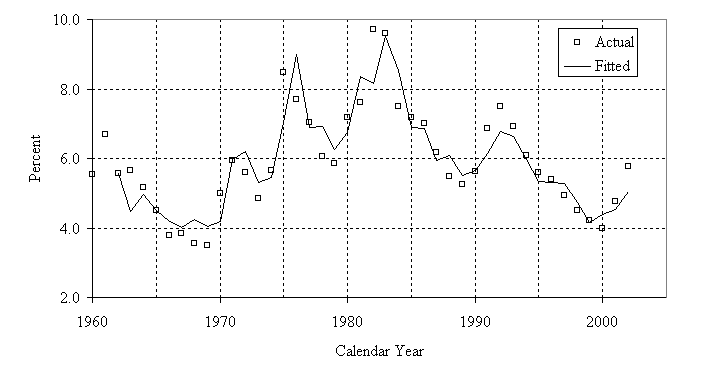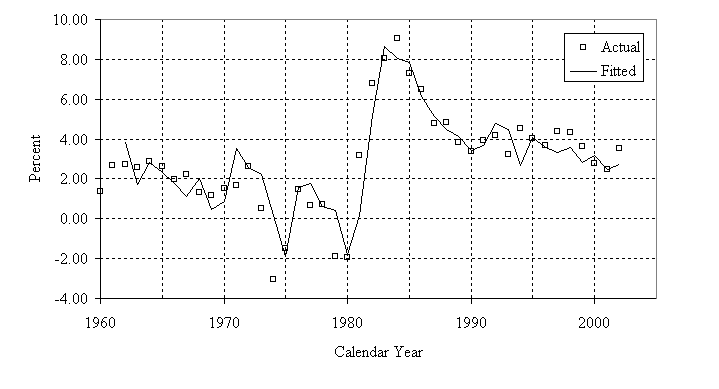## A Stochastic Model of the Long-Range Financial Status of the OASDI Program—September 2004

II. EQUATION SELECTION AND PARAMETER ESTIMATION

### D. RELATED ECONOMIC VARIABLES

The unemployment rate, inflation rate and real interest rate are simulated together using a vector autoregression, in order to capture the relationship among the three variables that economic theory suggests are related.1 In the vector autoregression, each variable is regressed on the prior-period values of all three variables. Vector autoregressions of different prior-period lengths were tested and it was determined that a vector autoregression including 2 prior years provided a reasonable fit. The historical period considered was 1960 to 2002.

For the vector autoregression, the unemployment rates (as defined in section II.D.1) were expressed as log-odds ratios2 to bound the values between 0 and 100 percent. In addition, the adjusted inflation rates (as defined in section II.D.2) had a logarithmic transformation3 applied to give them a lower bound for the vector autoregression. Instead of simply log-transforming the inflation rate series, 3.0 percent was added to the inflation rate series prior to the log-transformation. This gave the inflation rates a lower bound of -3.0 percent. For the remainder of this section and for section II.E, references to the unemployment rates and inflation rates refer to the transformed rates.

### 1. Unemployment Rate

The unemployment rate is the number of unemployed persons seeking work as a percentage of the civilian labor force. Historical values are published by the Bureau of Labor Statistics (BLS).4 The annual levels are an average of the 12 monthly rates.

Between 1960 and 1974, the unemployment rate was relatively stable, ranging from a low of 3.5 percent in 1969 to a high of 6.7 percent in 1961. Between 1975 and 1994, the unemployment rate moved to higher levels, and peaked at 9.7 percent in 1982. From 1994 to 2000, a rapid economic expansion resulted in unemployment rates falling to 4.0 percent.

For the unemployment rate equation, the R-squared value was 0.85. The actual and fitted values are shown in figure II.3. The modified equation is:

Ut = UtTR +0.96ut-1 −0.30ut-2 +0.40it-1 −0.08it-2 +0.75rt-1 +0.61rt-2 + ε1t .      (6)

In this equation, Ut represents the unemployment rate in year tUtTR is the unemployment rate from the TR04II in year t; ut represents the deviation of the unemployment rate from the TR04II unemployment rate in year tit is the deviation of the inflation rate from the TR04II inflation rate in year trt is the deviation of the real interest rate from the TR04II real interest rate in year t; and ε1t is the random error in year t.[D]

### 2. Inflation Rate

The inflation rate is defined as the annual growth rate in the Consumer Price Index for Urban Wage Earners and Clerical Workers (CPI). The BLS publishes historical values for the CPI.5 The BLS periodically introduces improvements to the CPI that affect its annual growth rate but does not revise earlier values. Consequently, OCACT has adjusted the CPI by back-casting the effects of the improvements on earlier values to improve consistency. The inflation rate is important because it determines the annual cost-of-living adjustment (COLA) for OASDI benefits.

Over the historical period from 1960 to 2002, the adjusted inflation rate ranged from a low of 0.8 percent in 1961 and 1962 to a high of 10.9 percent in 1980, and was 1.4 percent in 2002.

For the inflation rate equation, the R-squared value was 0.83. The actual and fitted values are shown in figure II.4. The modified equation is:

It = ItTR −0.77ut-1 +0.72ut-2 +0.60it-1 +0.30it-2 −4.85rt-1 +1.80rt-2 + ε2t .      (7)

In this equation, It is the CPI inflation rate in year tItTR is the CPI inflation rate from the TR04II in year tut represents the deviation of the unemployment rate from the TR04II unemployment rate in year tit is the deviation of the inflation rate from the TR04II inflation rate in year trt is the deviation of the real interest rate from the TR04II real interest rate in year t; and ε2t is the random error in year t.[D]

### 3. Real Interest Rate

All securities held by the OASI and DI Trust Funds are issued by the Federal Government. Almost all of these securities are special issues (i.e., securities issued only to the trust funds). Historical data on actual nominal interest rates of new purchases of these securities are published by OCACT.6 The nominal interest rate on new purchases of these securities for a given month is set equal to the average market yield on all marketable Federal obligations that are not callable and do not mature within the next 4 years.7 Annual nominal interest rates are the average of the 12 monthly rates, which in practice are compounded semiannually.8 The real interest rate earned on these obligations is equal to the annual (compounded) nominal yield divided by the inflation rate.

Looking at the period from 1960 to 2002, real interest rates on new purchases of special issues rose to much higher levels in the 1980s, as investors demanded higher risk premiums for increased uncertainty surrounding the unexpectedly high rates of inflation. Since then, the rate of inflation and the real interest rate have declined.

For the real interest rate equation, the R-squared value was 0.81. The actual and fitted values are shown in figure II.5. The modified equation is:

Rt = RtTR +0.06ut-1 −0.05ut-2 +0.03it-1 −0.03it-2 +1.23rt-1 −0.32rt-2 + ε3t .     (8)

In this equation, Rt represents the real interest rate in year tRtTR represents the real interest rate from the TR04II in year tut represents the deviation of the unemployment rate from the TR04II unemployment rate in year tit represents the deviation of the inflation rate from the TR04II inflation rate in year trt represents the deviation of the real interest rate from the TR04II real interest rate in year t; and ε3t is the random error term in year t.[D]

### Footnotes—

1 Foster (1994) suggested that a multivariate approach might capture a more appropriate range of variability for these economic variables. CBO implemented this approach in their stochastic model.

2 Ut = log[RUt / (1 − RUt)],where RUt is the unemployment rate in year t expressed as a decimal.

3 It = log(πt + 0.03),where πt is the percent change in the adjusted inflation rate in year t expressed as decimals.

4 www.bls.gov/cps/home.htm

5 www.bls.gov/cpi

7 For more details on the history of trust fund investment policy, see Actuarial Note 142, Social Security Trust Fund Investment Policies and Practices, by Jeff Kunkel, www.socialsecurity.gov/OACT/NOTES/note142.html.

8 For example, the annualized nominal yield on a special issue with a 6.0-percent nominal interest rate is equal to
(1 + .06 ⁄ 2)2 − 1 = 6.09 percent.